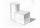# Diagonal + cylinder - math problems

#### Number of problems found: 7

• Surface area of the topA cylinder is three times as high as it is wide. The length of the cylinder’s diagonal is 20 cm. Find the surface area of the top of the cylinder.
• Axial sectionAxial section of the cylinder has a diagonal 40 cm. The size of the shell and the base surface are in the ratio 3:2. Calculate the volume and surface area of this cylinder.
• Axial sectionThe axial section of the cylinder has a diagonal 31 cm long, and we know that the area of the side and the base area is in ratio 3:2. Calculate the height and radius of the cylinder base.
• Surface area of cylinderDetermine the lateral surface of the rotary cylinder which is circumscribed cube with edge length 5 cm.
• Axial cut of a rectangleCalculate the volume and surface of the cylinder whose axial cut is a rectangle 15 cm wide with a diagonal of 25 cm long.
• Hexagon rotationA regular hexagon of side 6 cm is rotated through 60° along a line passing through its longest diagonal. What is the volume of the figure thus generated?
• Two ballsTwo balls, one 8cm in radius and the other 6cm in radius, are placed in a cylindrical plastic container 10cm in radius. Find the volume of water necessary to cover them.

We apologize, but in this category are not a lot of examples.
Do you have an interesting mathematical word problem that you can't solve it? Submit a math problem, and we can try to solve it.

We will send a solution to your e-mail address. Solved examples are also published here. Please enter the e-mail correctly and check whether you don't have a full mailbox.

Please do not submit problems from current active competitions such as Mathematical Olympiad, correspondence seminars etc...

Diagonal - math word problems. Cylinder Problems.